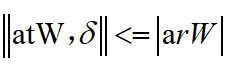# How to express it by using CVX? It is a SOCP problem (Moderator note: it is not an SOCP)

Hi, I want to use CVX to solve this problem:The first and second constraints are SOCP constraints.
My Matlab codes are as follow:
cvx_begin
variable W(2Nt)
minimize norm(W)
subject to
norm([at
W;theta]) <= abs(arW)sqrt(1/Tr);
norm([br
W;theta])<=abs(bt
W)*sqrt(1/Tt);
cvx_end
But it is wrong.
I hope someone can help me.Thanks!this is not even convex, so it can not be a socp.

Constraint is equivalent to